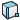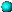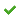# Plotting the Angular Displacement, Velocity, or Acceleration of a Part

You can plot the angular displacement, velocity, or acceleration of a part as it moves relative to another part or relative to the assembly origin.

1. From a Motion Analysis study, click Results and Plots(MotionManager toolbar).
2. In the PropertyManager under Result, for Category, select Displacement/Velocity/Acceleration.
3. For Subcategory, select one of the following:
• Angular Displacement
• Angular Velocity
• Angular Acceleration
4. For Select result component, select an option:
• Magnitude to resolve the magnitude in the direction of the result vector with respect to global coordinates.
• X Component, Y Component, or Z Component to calculate the magnitude of the result projected to the selected direction with respect to global coordinates.
X Component, Y Component, or Z Component options are not available for Angular Displacement.
5. Select features:
• Select a rotary motor to calculate the result for the angular motion of the location point for the motor.
• Select a mate to calculate the result of the relative angular motion of the geometric center of the first entity defining the mate with respect to the geometric center of the second entity defining the mate. The result is computed with respect to the global coordinates unless you select X Component, Y Component, or Z Component for the result component.
• Select three noncollinear points to an angle that specifies an angular displacement result, which calculates the motion of the angle as the points move with the assembly. Select the first two points on separate parts and the third point to specify the initial angle between the points.• Select a part face to calculate the result for the angular motion of the part face.
• Select two part faces to calculate the result for relative angular motion of the first selected part face relative to the frame defined by the second selected part face.
Selected part origins are indicated with a small ball.
6. Select the Plot Results options you require, and click.
The result appears in the MotionManager tree.
7. Click Calculate(MotionManager toolbar).